Next: Worked example 12.6: Launch Up: Orbital motion Previous: Worked example 12.4: Halley's

## Worked example 12.5: Mass of star

Question: A planet is in circular orbit around a star. The period and radius of the orbit are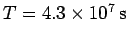and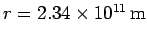, respectively. Calculate the mass of the star.

Answer: Letbe the planet's orbital angular velocity. The planet accelerates towards the star with acceleration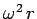. The acceleration due to the star's gravitational attraction is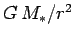, where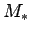is the mass of the star. Equating these accelerations, we obtain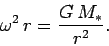Now,Hence, combining the previous two expressions, we get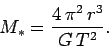Thus, the mass of the star is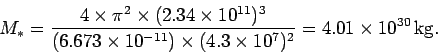Richard Fitzpatrick 2006-02-02